Test: Semiconductor Physics

# Test: Semiconductor Physics

Test Description

## 10 Questions MCQ Test GATE Electrical Engineering (EE) 2023 Mock Test Series | Test: Semiconductor Physics

Test: Semiconductor Physics for Electronics and Communication Engineering (ECE) 2023 is part of GATE Electrical Engineering (EE) 2023 Mock Test Series preparation. The Test: Semiconductor Physics questions and answers have been prepared according to the Electronics and Communication Engineering (ECE) exam syllabus.The Test: Semiconductor Physics MCQs are made for Electronics and Communication Engineering (ECE) 2023 Exam. Find important definitions, questions, notes, meanings, examples, exercises, MCQs and online tests for Test: Semiconductor Physics below.
Solutions of Test: Semiconductor Physics questions in English are available as part of our GATE Electrical Engineering (EE) 2023 Mock Test Series for Electronics and Communication Engineering (ECE) & Test: Semiconductor Physics solutions in Hindi for GATE Electrical Engineering (EE) 2023 Mock Test Series course. Download more important topics, notes, lectures and mock test series for Electronics and Communication Engineering (ECE) Exam by signing up for free. Attempt Test: Semiconductor Physics | 10 questions in 30 minutes | Mock test for Electronics and Communication Engineering (ECE) preparation | Free important questions MCQ to study GATE Electrical Engineering (EE) 2023 Mock Test Series for Electronics and Communication Engineering (ECE) Exam | Download free PDF with solutions
 1 Crore+ students have signed up on EduRev. Have you?
Test: Semiconductor Physics - Question 1

### The mobility of free electrons and holes in pure germanium are 3800 and 1800 cm2/V-s respectively. The corresponding values for pure silicon are 1300 and 500 cm2/V-s, respectively. Assuming ni = 2.5 x 1013 cm-3 for germanium and ni = 1.5 x 1010 cm-3 for silicon at room temperature, the values of intrinsic conductivity for germanium and silicon are respectively given by

Detailed Solution for Test: Semiconductor Physics - Question 1

The intrinsic conductivity for germanium is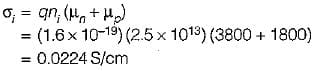The intrinsic conductivity for silicon is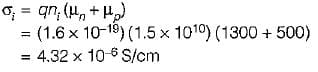Test: Semiconductor Physics - Question 2

### What is the concentration of holes and electrons in n-type Silicon at 300°K, if the conductivity is 30 S/cm? Assume at 300°K, ni = 1.5 x 1010/cm3, μn = 1300 cm2/V-s and μp= 500 cm2/V-s

Detailed Solution for Test: Semiconductor Physics - Question 2

The conductivity of an n-type silicon is a σ = qn μn.
Concentration of electrons,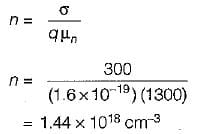Using “mass-action law'',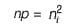∴ Concentration of holes,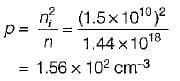Test: Semiconductor Physics - Question 3

### When an electric field is applied across a semiconductor, free electrons in it will accelerate due to the applied field, and gain energy. This energy can be lost as heat when the electrons

Detailed Solution for Test: Semiconductor Physics - Question 3

When an electron is accelerated by the potential applied to a semiconductor, the energy gained from the field may then be transferred to an atom when the electron collides with the atom.

Test: Semiconductor Physics - Question 4

If a current of 1.6 μA is flowing through a conductor, the number of electrons crossing a particular cross-section per second will be

Detailed Solution for Test: Semiconductor Physics - Question 4

Given, I = 1.6 x 10-6 A
= 1.6 x 10-6 Coulomb/second
Charge crossing a particular cross-section per second = 1.6 x 10-6 C Hence, number of electrons crossing a particular cross-section per second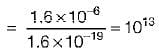Test: Semiconductor Physics - Question 5

Match List-l with List-lI and select the correct answer using the codes given below the lists: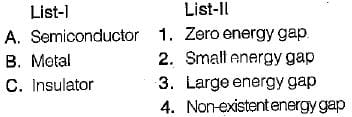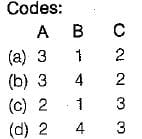Detailed Solution for Test: Semiconductor Physics - Question 5

Energy band diagram for semiconductor, metal and insulator are shown below.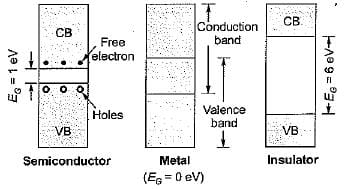Test: Semiconductor Physics - Question 6

If elements in column IV of the periodic table are placed in increasing order of their atomic number, the order will be

Test: Semiconductor Physics - Question 7

Assertion (A): The drift velocity is in the direction opposite to that of the electric field.
Reason (R): At each inelastic collision with an ion, an electron loses energy, and a steady-state condition is reached where a finite value of drift speed is attained.

Detailed Solution for Test: Semiconductor Physics - Question 7

Both assertion and reason are individually correct statements. However, the reason for assertion is that due to the applied electric field, and electrostatic force is developed on the electron and the electrons would be accelerated in a direction opposite to the applied electric field and this motion is called directed motion of electron.

Test: Semiconductor Physics - Question 8

The density and mobility of electrons in a conductor are respectively 1020/cm3 and 800 cm2/V-s. If a uniform electric field of 1 V/cm exists across this conductor, then the electron current density would be approximately

Detailed Solution for Test: Semiconductor Physics - Question 8

The current density is given by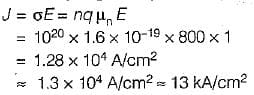Test: Semiconductor Physics - Question 9

A semiconductor is doped with a donor density ND and no acceptors. If the intrinsic concentration is ni then the free electron density(n) will be equal to

Detailed Solution for Test: Semiconductor Physics - Question 9

Given, NA = 0
Using “charge neutrality equation”, we have:
ND + P = NA + n
or, ND + p = ....(i)
Using'“Mass-action law”, we have: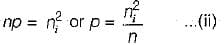Substituting value of p from equation (ii) in equation (i), we have: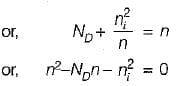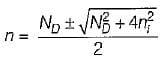Neglecting negative sign, we get: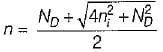= free electron density or concentration

Test: Semiconductor Physics - Question 10

In a p-type semiconductor, p = 1016/cm2, and μp = 400 cm2/V-s. If a magnetic field (B) of 5 x 10-4 Weber/cm2 is applied in the x-direction, and an electric field of 2000 V/m is applied in +y direction. The value of electric field caused due to the “Hall effect” is

Detailed Solution for Test: Semiconductor Physics - Question 10

The force acting on a charge q placed in a magnetic field B and an electric field E is given by
F = qv x B
The velocity of the hole placed in an electric field is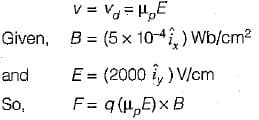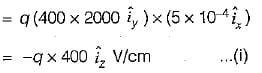Now, F - + q E ...(ii) (+q = charge on a hole)
Comparing equations (i) and (ii), the electric field due to the Hall effect will be -400 V/cm in +z direction.

## GATE Electrical Engineering (EE) 2023 Mock Test Series

21 docs|269 tests
 Use Code STAYHOME200 and get INR 200 additional OFF Use Coupon Code
Information about Test: Semiconductor Physics Page
In this test you can find the Exam questions for Test: Semiconductor Physics solved & explained in the simplest way possible. Besides giving Questions and answers for Test: Semiconductor Physics, EduRev gives you an ample number of Online tests for practice

## GATE Electrical Engineering (EE) 2023 Mock Test Series

21 docs|269 tests